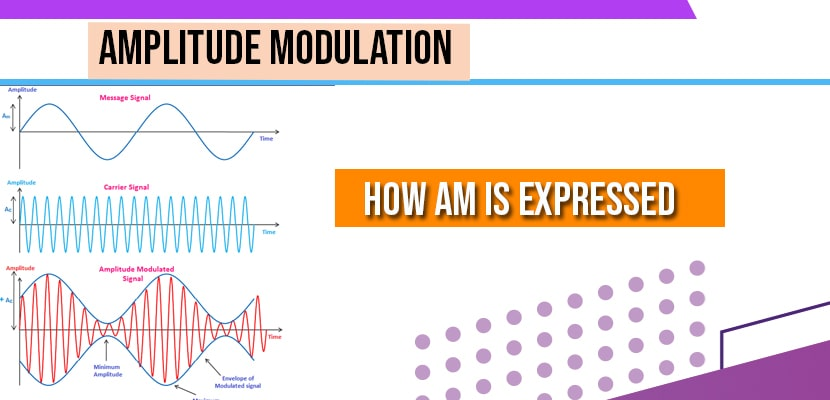# Amplitude Modulation

In communication a carrier signal is a sinusoidal signal denoted by

C(t)=Ac Cos(2ᴨfct)

Ac= Amplitude of the carrier

C(t)= carrier signal

fc= carrier frequency

This carrier signal is fundamental in any communication system message signal or baseband signal is modulated. There are many ways to modulate the signal one among them is Amplitude modulation. In Amplitude modulation the carrier signal is modulated buy message signal or baseband signal. Or in other way Amplitude modulation is a process in which the amplitude of the carrier signal is varied instantaneously in accordance with the baseband signal keeping phase and frequency constant.

## How Amplitude Modulation is Expressed

AM signal is expressed as:

ka= sensitivity of the AM signal

m(t)=message to be transmitted

In amplitude modulation what actually happens is that we have message signal which has the message to be transmitted and also carrier signal, when we multiply this carrier signal; by message the amplitude of the carrier which is constant varies according to the message.